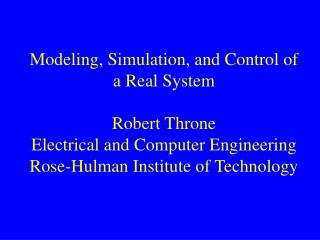Download PresentationModeling, Simulation, and Control of a Real System Robert Throne Electrical and Computer Engineering Rose-Hulman Institu

# Modeling, Simulation, and Control of a Real System Robert Throne Electrical and Computer Engineering Rose-Hulman Institu - PowerPoint PPT PresentationDownload Presentation## Modeling, Simulation, and Control of a Real System Robert Throne Electrical and Computer Engineering Rose-Hulman Institu

- - - - - - - - - - - - - - - - - - - - - - - - - - - E N D - - - - - - - - - - - - - - - - - - - - - - - - - - -
##### Presentation Transcript

1. Modeling, Simulation, and Control of a Real SystemRobert ThroneElectrical and Computer EngineeringRose-Hulman Institute of Technology

2. Introduction • Models of physical systems are widely used in undergraduate science and engineering education. • Students erroneously believe even simple models are exact.

3. Introduction • Obtained ECP Model 210a rectilinear mass, spring, damper systems for use in both system dynamics and controls systems labs. • Models for these systems are easy to develop and students have seen these types of models in a variety of courses.

4. Introduction(mass, springs, and encoder)

5. Introduction(motor, rack and pinion, damper, and spring connecting to first cart)

6. Introduction We developed four groups of labs for the ECE introductory controls class for a one degree of freedom system: • Time domain system identification. • Frequency domain system identification. • Closed loop plant gain estimation. • Controller design based on the model.

7. Parameters to Identify In the transfer function model we need to determine • the gain • the damping ratio • the natural frequency

8. Time Domain System Identification • Log decrement analysis • Fitting the step response of a second order system to the measured step response

9. Frequency Domain System Identification • Determine steady state frequency response by exciting the system at different frequencies. • Compare to predicted frequency response. • Optimize transfer function model to best fit measured frequency response.

10. Model/Actual Frequency Response(from log-decrement)

11. Model/Actual Frequency Response(from fitting step response)

12. Model From Frequency Response

13. Closed Loop Plant Gain Estimation • We model the motor as a gain, , and assume it is part of the plant • We use a proportional controller with gain • The closed loop system is • The closed loop plant gain is then

14. Closed Loop Plant Gain Estimation • Input step of amplitude A • Steady state output • The closed loop plant gain is given by

15. Results with Controllers After identifying the system, I, PI, PD, and PID controllers were designed using Matlab’s sisotool to control the position of the mass (the first cart). Both predicted (model based) responses and actual (real system) responses are plotted on the same graph.

16. Integral (I) Controller

17. ``It doesn’t work!’’

18. Proportional+Derivative (PD) Controller

19. PID Controller(complex conjugate zeros)

20. PID Controller(real zeros)

21. State Variable Feedback

22. Conclusions Students learn: • Simple, commonly used models are not exact, but still very useful. • Simple models are a reasonable starting point for design. • Motors have limitations which must be incorporated into designs.

23. Conclusions We have extended these labs to include Model matching • ITAE • quadratic optimal • polynomial equation (Diophantine) 2 and 3 DOF state variable models

24. Acknowledgement This material is based upon work supported by the National Science Foundation under Grant No. 0310445